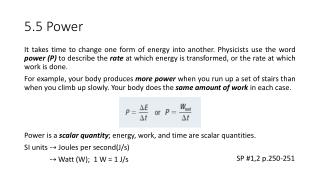DownloadDownload Presentation5.5 Power

# 5.5 Power

Download Presentation## 5.5 Power

- - - - - - - - - - - - - - - - - - - - - - - - - - - E N D - - - - - - - - - - - - - - - - - - - - - - - - - - -
##### Presentation Transcript

1. 5.5 Power It takes time to change one form of energy into another. Physicists use the word power (P) to describe the rate at which energy is transformed, or the rate at which work is done. For example, your body produces more power when you run up a set of stairs than when you climb up slowly. Your body does the same amount of work in each case. Power is a scalar quantity; energy, work, and time are scalar quantities. SI units ⤍Joules per second(J/s) ⤍ Watt (W); 1 W = 1 J/s SP #1,2 p.250-251

2. 5.5 Electrical Power Electrical devices transform electrical energy into other forms of energy. When electrical devices transform energy more quickly, they are more powerful, and when they transform energy more slowly, they are less powerful. The maximum power of an electric device or appliance is referred to as the device’s power rating. Power ratings of electrical devices tend to be in the hundreds or thousands of watts. SP #1 p.252

3. 5.5 Cost of Electricity Companies that provide electrical energy to consumers measure the amount of electrical energy used through electricity meters.This amount depends on the number of devices being used, the power rating of each device, and the total time of use for each device. Electricity meters measure electrical energy used in units of kilowatthours (kWh). Rearranging the power equation for energy yields ∆E = P∆t, where the product of power, P, measured in kilowatts (kW), and the time interval, ∆t, measured in hours, produces the unit kilowatthours (kWh). SP #1 p.253

4. 5.5 Homework • Practice # 1,2 p.251 • Practice # 1 p.252 • Practice # 1 p.253 • Questions # 1-4 p.254